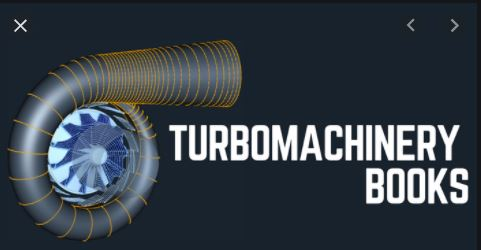## turbomachinery notes pdf |turbomachinery lecture notes | turbomachinery notes pdf | turbo machine handwritten notes | turbomachine pdf notes|Handwritten notes Mechanical Engineering | Written by Ashish SirTurbomachinery, in mechanical engineering, describes machines that transfer energy between a rotor and a fluid, including both turbines and compressors. While a turbine transfers energy from a fluid to a rotor, a compressor transfers energy from a rotor to a fluid.

## Turbomachinery notes Syllabus

Unit I: Energy transfer in turbo machines: application of first and second laws of thermodynamics to turbo machines, moment of momentum equation and Euler turbine equation, principles of impulse and reaction machines, degree of reaction, energy equation for relative velocities, one dimensional analysis only.

Unit II: Steam turbines: impulse staging, velocity and pressure compounding, utilization factor, analysis for optimum U.F Curtis stage, and Rateau stage, include qualitative analysis, effect of blade and nozzle losses on vane efficiency, stage efficiency, analysis for optimum efficiency, mass flow and blade height. Reactions staging: Parson’s stages, degree of reaction, nozzle efficiency, velocity coefficient, stator efficiency, carry over efficiency, stage efficiency, vane efficiency, conditions for optimum efficiency, speed ratio, axial thrust, reheat factor in turbines, problem of radial equilibrium, free and forced vortex types of flow, flow with constant reaction, governing and performance characteristics of steam turbines.

Unit III: Water turbines: Classification, Pelton, Francis and Kaplan turbines, vector diagrams and work-done, draft tubes, governing of water turbines. Centrifugal Pumps: classification, advantage over reciprocating type, definition of mano-metric head, gross head, static head, vector diagram and work done. Performance and characteristics: Application of dimensional analysis and similarity to water turbines and centrifugal pumps, unit and specific quantities, selection of machines, Hydraulic, volumetric, mechanical and overall efficiencies, Main and operating characteristics of the machines, cavitations.

Unit IV: Rotary Fans, Blowers and Compressors: Classification based on pressure rise, centrifugal and axial flow machines. Centrifugal Blowers Vane shape, velocity triangle, degree of reactions, slip coefficient, size and speed of machine, vane shape and stresses, efficiency, characteristics, fan laws and characteristics. Centrifugal Compressor – Vector diagrams, work done, temp and pressure ratio, slip factor, work input factor, pressure coefficient, Dimensions of inlet eye, impeller and diffuser. Axial flow Compressors- Vector diagrams, work done factor, temp and pressure ratio, degree of reaction, Dimensional Analysis, Characteristics, surging, Polytrophic and isentropic efficiencies.

Unit V: Power Transmitting turbo machines: Application and general theory, their torque ratio, speed ratio, slip and efficiency, velocity diagrams, fluid coupling and Torque converter, W.E.F. July 2017 Academic Session 2017-18 characteristics, Positive displacement machines and turbo machines, their distinction. Positive displacement pumps with fixed and variable displacements, Hydrostatic systems hydraulic intensifier, accumulator, press and crane.

## ChAPTER-1

Unit I: Energy transfer in turbo machines: application of first and second laws of thermodynamics to turbo machines, moment of momentum equation and Euler turbine equation, principles of impulse and reaction machines, degree of reaction, energy equation for relative velocities, one dimensional analysis only.